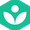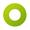## Analyzing relationships between variables using tables and equations | 6th grade | Khan Academy

Titel:
Analyzing relationships between variables using tables and equations | 6th grade | Khan Academy
Beschreibung:

Sal shows an example where, given a context/graph, we connect a table and equation to analyze the relationship between the variables in the context.

more » « less
Video Language:
English
Team:Khan Academy
Duration:
02:49Amara Bot edited Englisch subtitles for Analyzing relationships between variables using tables and equations | 6th grade | Khan Academy Somebody (possibly automatically) added a video: Analyzing relationships between variables using tables and equations | 6th grade | Khan Academy